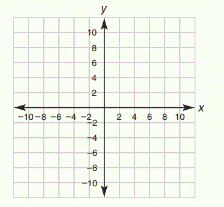Chapter 10.CT, Problem 6CTElementary Geometry For College St...

7th Edition
Alexander + 2 others
ISBN: 9781337614085

Solutions

Chapter
SectionElementary Geometry For College St...

7th Edition
Alexander + 2 others
ISBN: 9781337614085
Textbook Problem

Using the table from Exercise 5, sketch the graph of 2 x + 3 y = 12 .To determine

To draw:

The graph of the equation 2x+3y=12

Explanation

To draw a graph for a equation,

First we need to find the x-intercept and y-intercept.

For finding the x-intercept, make y as 0 and solve for x in the given equation.

For finding the y-intercept, make x as 0 and solve for y in the given equation.

Substitute x=0 in the given equation 2x+3y=12,

20+3y=12

On simplifying,

3y=12

The coefficient of y is 3, thus divide both sides by 3.

3y3=123

y=4

Thus the first point is 0, 4.

Substitute x=3 in the given equation 2x+3y=12,

23+3y=12

On simplifying,

6+3y=12

3y=12-6

3y=6

The coefficient of y is 3, thus divide both sides by 3.

3y3=63

y=2

Thus the second point is 3, 2.

Substitute y=4 in the given equation 2x+3y=12,

2x+34=12

On simplifying,

2x+12=12

2x=12-12

2x=0

x=0

Thus the third point is 0, 4.

Substitute x=9 in the given equation 2x+3y=12,

29+3y=12

On simplifying,

18+3y=12

3y=12-18

3y=-6

The coefficient of y is 3, thus divide both sides by 3

Still sussing out bartleby?

Check out a sample textbook solution.

See a sample solution

The Solution to Your Study Problems

Bartleby provides explanations to thousands of textbook problems written by our experts, many with advanced degrees!

Get Started

Find more solutions based on key concepts